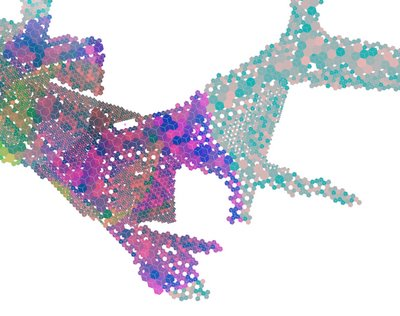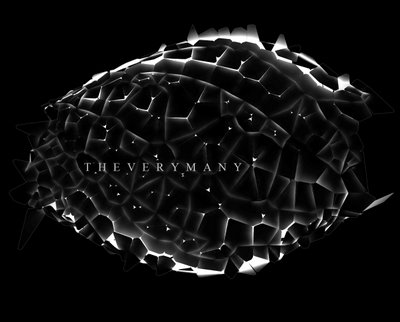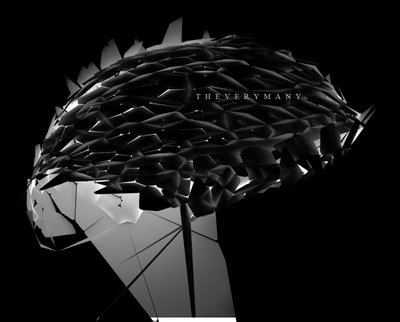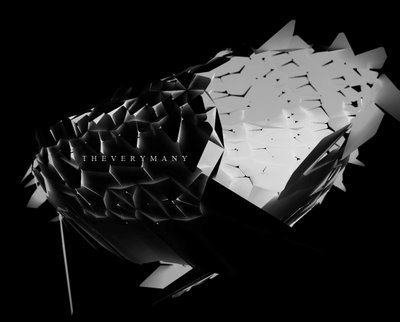#### MARC FORNES & THEVERYMANY™

PRACTICING AT THE INTERSECTION OF ART + ARCHITECTURE ^ COMPUTATION

## 070530_PatternFirst test looking at pattern for the floor of an hotel lobby (SOM Interior); one continuous circulation space is treated as a blend of different “moments” or sub-spaces highlighted here through an overall mapping system of ceramic tiles.

THE PATTERN (ie : Wikipedia.org)

The PATTERN is a form, template, or model (or, more abstractly, a set of rules) which can be used to make or to generate things or parts of a thing, especially if the things that are created have enough in common for the underlying pattern to be inferred, in which case the things are said to exhibit the pattern. Pattern matching is the act of checking for the presence of the constituents of a pattern. The detection of underlying patterns is called pattern recognition. The question of how different patterns emerge is accomplished through the work of the scientific field of pattern formation. Patterns are also related to repeated shapes or objects, sometimes referred to as elements of the series.

The simplest patterns are based on repetition/PERIODICITY: several copies of a single template are combined without modification.”INCREASING THE RESOLUTION: variation criteria are illustrated through the repetition of “identical”, “similar” or “self-similar” hexagonal components via changes of scale (each tile format can be recomposed by an assembly of smaller tiles having the same shape), color (allowed within “stepped ranges” in order not to maximize the number of different colors), finishes ( from glossy to mat and ruff) and orientation (the poly-directionality of the six sided shape of the hexagon chosen here for its best fit to the numerous branching direction of the project is reduced via a re-subdivision into three pairs of two sides, shaping some sort of arrow acting as a compass)

Pseudo Code:

post in progress – requires editing…

## 101 – test

www.theverymany.net

## 070519_Computational_GeometryCOMPUTATIONAL GEOMETRY (from Wikipedia.org)
– readings while testing VRay fo rhino4 –

In computer science, computational geometry is the study of algorithms to solve problems stated in terms of geometry. Some purely geometrical problems arise out of the study of computational geometric algorithms, and the study of such problems is also considered to be part of computational geometry.

The main impetus for the development of computational geometry as a discipline was progress in computer graphics, computer-aided design and manufacturing (CAD/CAM), but many problems in computational geometry are classical in nature.

Other important applications of computational geometry include robotics (motion planning and visibility problems), geographic information systems (GIS) (geometrical location and search, route planning), integrated circuit design (IC geometry design and verification), computer-aided engineering (CAE) (programming of numerically controlled (NC) machines).

The main branches of computational geometry are:

– COMBINATORIAL COMPUTATIONAL GEOMETRY, also called algorithmic geometry, which deals with geometric objects as discrete entities. A groundlaying book in the subject by Preparata and Shamos dates the first use of the term “computational geometry” in this sense by 1975
– NUMERICAL COMPUTATIONAL GEOMETRY, also called machine geometry, computer-aided geometric design (CAGD), or geometric modeling, which deals primarily with representing real-world objects in forms suitable for computer computations in CAD /CAM systems. This branch may be seen as a further development of descriptive geometry and is often considered a branch of computer graphics and/or CAD. The term “computational geometry” in this meaning has been in use since 1971/// COMBINATORIAL COMPUTATIONAL GEOMETRY ///

The primary goal of research in combinatorial computational geometry is to develop efficient algorithms and data structures for solving problems stated in terms of basic geometrical objects: points, line segments, polygons, polyhedra, etc.

Some of these problems seem so simple that they were not regarded as problems at all until the advent of computers. Consider, for example, the Closest pair problem:

Given n points in the plane, find the two with the smallest distance from each other.
One could compute the distances between all the pairs of points, of which there are n(n-1)/2, then pick the pair with the smallest distance. This brute-force algorithm takes O(n2) time; i.e. its execution time is proportional to the square of the number of points. A classic result in computational geometry was the formulation of an algorithm that takes O(n log n). Randomized algorithms that take O(n) expected time, as well as a deterministic algorithm that takes O(n log log n) time, have also been discovered.

For modern GIS, computer graphics, and integrated-circuit design systems routinely handling tens and hundreds of million points, the difference between O(n2) and O(n log n) can be the difference between days and seconds of computation. Hence the emphasis on computational complexity in computational geometry.

PROBLEM CLASSES
The core problems in computational geometry may be classified in different ways, according to various criteria. The following general classes may be distinguished.

STATIC PROBLEMS
In the problems of this category, some input is given and the corresponding output needs to be constructed or found. Some fundamental problems of this type are:

– Convex hull: Given a set of points, find the smallest convex polygon containing all the points.
– Line segment intersection: Find the intersections between a given set of lines.
– Delaunay triangulation: Connect a given set of points forming triangles that satisfy some fatness properties.
– Voronoi diagram: Given a set of points, partition the space according to which point is closest.
– Linear programming: Given a set of halfspaces, find the bottommost point contained in their intersection.
– Closest pair of points: Given a set of points, find the two with the smallest distance from each other.
– Euclidean shortest path: Connect two points in a Euclidean space (with polyhedral obstacles) by a shortest path.
– Polygon triangulation: Given a polygon, partition its interior by connecting its vertices.
The computational complexity for this class of problems is estimated by the time and space (computer memory) required to solve a given problem instance.

GEOMETRIC QUERY PROBLEMS
In geometric query problems, commonly known as geometric search problems, the input consists of tho parts: the invariant part (called search space) and the query part, which varies over the problem instances. The invariant part typically needs to be preprocessed, in a way that multiple queries can be answered efficiently. Sometimes, other operation, like the insertion and removal of points are also allowed. Some fundamental geometric query problems are:

– Range searching: Preprocess a set of points, in order to efficiently count the number of points inside a query region.
– Point location: Given a partitioning of the space into cells, produce a data structure that efficiently tells in which cell a query point is located.
– Nearest neighbor: Preprocess a set of points, in order to efficiently find which point is closest to a query point.
– Ray tracing: Given a set of objects in space, produce a data structure that efficiently tells which object a query ray intersects first.
The computational complexity for this class of problems is estimated by:

the time and space required to construct the data structure to be searched in
the time (and sometimes an extra space) to answer a queries.

DYNAMIC PROBLEMS
A yet another major class are the dynamic problems, in which the goal is to find an efficient algorithm for finding a solution repeatedly after each incremental modification of the search space (addition or deletion input geometric elements). Algorihms for problems of this type typically involve dynamic data structuress. Any of the computational geometric problems may be converted into a dynamic one. For example, the range searching problem may be converted into the dynamic range searching problem by providing for addition and/or deletion of the points.

The computational complexity for this class of problems is estimated by:

the time and space required to construct the data structure to be searched in
the time and space to modify the searched data structure after an incremental change in the search space
the time (and sometimes an extra space) to answer a queries.

VARIATIONS
Some problems may be treated as belonging to either of the categories, depending on the context. For example, consider the following problem.

– Point in polygon: Decide whether a point is inside or outside a given polygon.
In many applications this problem is treated as a single-shot one, i.e., belonging to the first class. For example, in many applications of computer graphics a common problem is to find which area on the screen is clicked by a mouse cursor. However in some applications the polygon in question is invariant, while the point represents a query. For example, the input polygon may represent a border of a country and a point is a position of an aircraft, and the problem is to determine whether the aircraft violated the border. Finally, in the previously mentioned example of computer graphics, in CAD applications the changing input data are often stored in dynamic data structures, which may be exploited to speed-up the point-in-polygon queries.

In some contexts of query problems there are reasonable expectations on the sequence of the queries, which may be exploited either for efficient data structures or for tighter computational complexity estimates. For example, in some cases it is important to know the worst case for the total time for the whole sequence of N queries, rather than for a single query./// NUMERICAL COMPUTATIONAL GEOMETRY ///

This branch is also known as GEOMETRIC MODELLING, computer-aided geometric design (CAGD), and may be often found under the keyword curves and surfaces.

Core problems are curve and surface modelling and representation.

The most important instruments here are parametric curves and parametric surfaces, such as Bezier curves, spline curves and surfaces. An important non-parametric approach is the level set method.

First (and still most important) application areas are shipbuilding, aircraft, and automotive industries. However because of modern ubiquity and power of computers even perfume bottles and shampoo dispensers are designed using techniques unheard of by shipbuilders of 1960s.

## 070502_MF | Tessellation / Flat_Panels v3.0

Some sort of panels “stability” mapping based on the number of connexions for each panels toits neighbours:
– GREEN: connexion to at least three neighbours
– YELLOW: connexion to two neighbours
– RED: connexion to one single neighbour